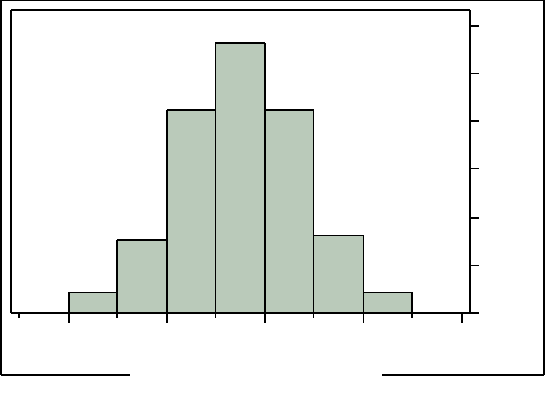Class Notes (1,100,000)
US (470,000)
ISU (1,000)
STAT (6)
Lecture 13

# STAT 101 Lecture Notes - Lecture 13: Standard Deviation, Underweight

Department
Statistics
Course Code
STAT 101
Professor
Stephenson Robert
Lecture
13

This preview shows page 1. to view the full 4 pages of the document.Statistics 104 - Laboratory 8
Normal Models
Often a population will have a variable whose distribution can be modeled using a normal
model with a population mean μ and a population standard deviation σ.
1. Market weight of gilts.
The market weight, in pounds, of 179 gilts, female hogs is displayed in the histogram
below.
10
20
30
40
50
Count
150 200 250 300 3
a) Describe the shape of the distribution. Why is a normal model a reasonable
model for the distribution of the population of gilt market weights?
b) Use a normal model for the population of gilt market weights with population
mean μ = 236.5 pounds and population standard deviation σ = 31.2 pounds to find
The probability that a gilt market weight will be less than 300 pounds.
The probability that a gilt market weight will be greater than 275 pounds.
The probability that a gilt market weight will be between 200 and 300
pounds.
The value such that 4% of all gilt market weights will be less than that
value.
The value such that 25% of all gilt market weights will be greater than that
value.
The values such that the middle 90% of all gilt market weights will fall
between those values.
Market Weight (lbs)
1